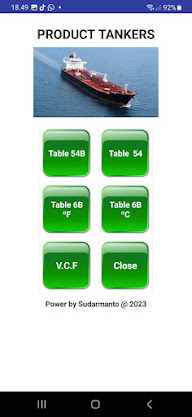## Senin, 01 April 2013

### BARGES DRAFT SURVEY PROCEDURE

BARGES DRAFT SURVEY  ( Draft  Survey Tongkang  )

Before we explain the following barges draft survey, we provide
simple application to calculate the barge draft survey.
Just calculate only, no print out.

Here is a draft survey barge display applications that can be

Here with provide application detail discharge calculation Barges 230 feet, 250 feet, 270 feet and 300 feet draft survey. with print out and compare BL Figure.

install component system 32

Contact : sudarmanto.clg@gmail.com

BARGES DRAFT SURVEY PROCEDURE

This Survey Procedure is International Standard for any type of barges.
APPARENT TRIM
The Forward (Fwd), Aft (Aft), and Mid (Mid) drafts are read at both Port (P) and
Starboard (Stbd) marks. The P and S readings are added, and the result divided by two.
The Aft draft is subtracted from the Fwd draft, and the result is Apparent Trim.
If Trim is positive, Barges is trimmed By the Head; if Trim is negative, Barge is

trimmed By the Stern.

Fwd Draft = Fwd(P) + Fwd(S)
2
Aft Draft = Aft(P) + Aft(s)
2
Mean Mid = Mid(P) + Mid(s)
2
Trim = Fwd – Aft

BARGES NO NEED DRAFT CORRECTIONS

MEAN DRAFT CORRECTION
The Quarter Mean ( QM ) or Mean Draft Corrected for  Deformation must be solved next.
Use the corrected draft values
First calculate the Fwd/Aft Mean Draft. Add Fwd to Aft, and divide the result by two:
Fwd /Aft Mean = Fwd + Aft
2
Next, calculate the Mean of Mean Add the Fwd/Aft  Mean to the Mid Mean, and divide the

result by two:
Mean of Mean = Fwd/Aft Mean + Mid Mean
2
Now calculate the QM Add the Mean of Mean  to the Mid Mean,
and divide the result by two
QM =  Mean of Mean +  Mid Mean
2
Refer to barges Hydrostatic Tables for the following values:
TPC:  tonnes per Centimetre Immersion
MTC: Moment to change Trim One Centimetre
Lcf:  Longitudinal centre of Flotation

Interpolation
Calculate the Displacement Correction ( DISP. Corr.)
1) Subtract the nearest smaller Draft from the calculated   QM.
2)Multiply the result by 100 to convert Meters to Centimetres.
3) Multiply this by the TPC for the displacement.
4) This correction is added to the displacement given  for   the nearest smaller draft.

Draft Remainder (cm) = Draft remainder x 100
DISP. Corr. =  TPC x Draft remainder (cm)
Displacement =  DISP. +  Corrected. = Actual Displacement

TRIM CORRECTION
Before calculating the First Trim Correction, Corrected Trim (CT)  must be converted
from meters to centimetres. Multiply CT (in) by 100 to get centi-metres.
To calculate the First Trim Correction, multiply TRIM by TPC, then multiply the product
by the longitudinal Centre of Flotation (LCF) x 100. Then, divide the final product
by the Length Between Perpendiculars (LBP).
CT = CT x 100
First Correction = TRIM x TPC x Lcf x 100
LBP
Second Correction = T2 +/-50 cm x MTC diff.
LBP

The first correction can be either positive (add), or negative (subtract), depending
on the location of the LCF  and the trim condition. (It's mean sign of Lcf and TRIM )
TRIMMED BY THE HEAD        Lcf is Forward (Fwd) (+) ADD Trim Correction
Lcf is Aft (Aft) (-) SUBTRACT Trim Correction
TRIMMED BY THE STERN       Lcf is Fwd (—) SUBTRACT Trim Correction
Lcf is Aft (+) ADD Trim Correction
The second  Trim  Correction is  required when the Trim is greater than the LBP
divided by 100. It may be applied without adverse effect at smaller trims.
The second correction is always (+) (additive) regardless of the trim or other factors.
Before calculating the Second Trim Correction, MTC differ-ence, sometimes referred
to as dM/dZ, must be found.
ADD 50 cm to the Quarter Mean Draft ( QM ) to  find the corresponding MTC from
barges Hydrostatic Tables .
SUBTRACT 50 cm from the Quarter Mean Draft (QM) to find the corresponding MTC
from barges Hydrostatic Tables ..

How to find MTC diff  in the Hydrostatic Tables :

a)  Q M + 50cm = MTC ( Found in barges Hydrostatic Tables .)
b)  Q M - 50cm = MTC ( Found in barges Hydrostatic Tables .)
MTC diff = ( a) — ( b)

a)     QM = 3.675
+ 0.50
4.175         MTC = 169.4
b)    QM = 3.675
- 0.50
3.175        MTC =160.7
(a)  =    169.4
(b) = - 160.7
MTC diff  =        8.7

SEA WATER SPECIFIC GRAVITY (SG) CORRECTION
A Specific Gravity (SG) of 1.025  is generally assumed
for SeaWater in calculating Displacement (DISP). Because the Sg. is almost never exactly
1.025, Sg. correction must be calculated.
Sg. is always minus if the measured Sg. is 1.025 or less.

Calculate the Sg. correction by subtracting the measured density from 1.025, divide
this by 1.025 and then multi-ply that answer by the DISP.
Sg. corr. = 1.025 - Measured Density x DISP.
1.025

FINAL SURVEY
The Final Survey follows the same procedure as the Initial Survey. Total cargo equals

DISP. minus Lightship Weight.

# See you at the next blog .....SHIP'S DRAFT SURVEY